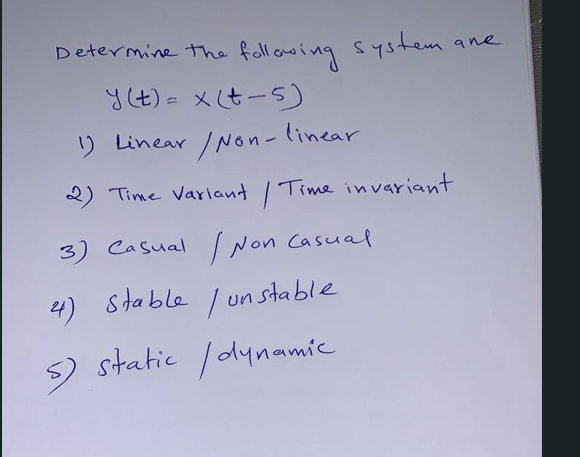Home / Expert Answers / Electrical Engineering / determine-the-following-system-ane-y-t-x-t-5-1-linear-non-linear-2-time-variant-time-pa928

# (Solved): Determine the following system ane \[ y(t)=x(t-5) \] 1) Linear/Non - linear 2) Time variant / Time ...Determine the following system ane \[ y(t)=x(t-5) \] 1) Linear/Non - linear 2) Time variant / Time invariant 3) Casual INon casual 4) Stable /unstable 5) Static /dynamic

We have an Answer from Expert